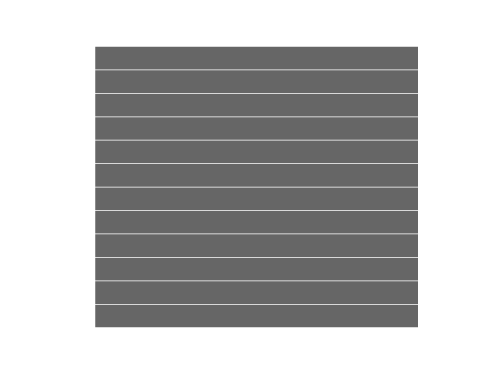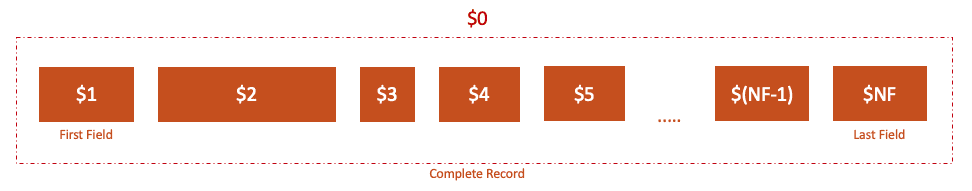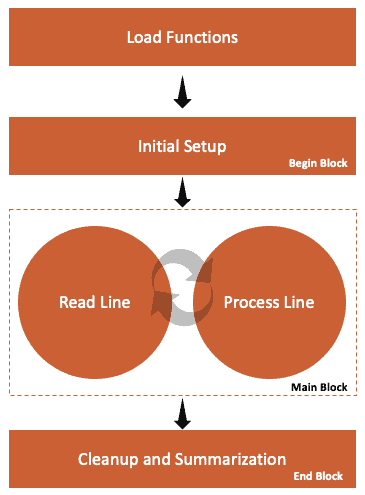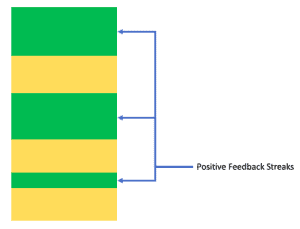## 1. Overview

Working with text is part of the daily routine of a lot of developers. In this tutorial, we’ll learn how we can process large text datasets using the AWK programming language.

## 2. Origin Story

Unless we know the origin story of AWK, we might end up misinterpreting its meaning. So, let’s time travel to the past and read the minds of AWK’s creators.

In the 1970s, Bell Labs focused heavily on delivering a range of phenomenal computing inventions, and it all started with the birth of the Unix operating system in the previous decade. To further empower the Unix users, they created software by picturing a toolbox wherein each tool would do one small task well.

Among others, one of the underlying computing needs was to process large text files efficiently. Neither the regular editors nor the existing programming languages could efficiently address this requirement. On the one hand, it was nearly impossible for a regular editor to even open large text files, while on the other hand, it was too much code to write, even for small tasks.

As we often say that necessity is the mother of invention, three computer scientists, namely Alfred Aho, Peter J. Weinberger, and Brian Kernighan, soon came up with a solution by co-authoring a new programming language. Further, they ended up naming it as AWK, by taking the first letter from each of their last names.

Even after so many decades, we can appreciate the beauty of this invention by the mere fact that it’s still relevant today in this fast-changing world of technology.

Using AWK, we can process large text files without worrying about their sizes. This is possible because instead of loading the entire text into memory, we’re reading it sequentially in chunks. Let’s see the AWK-way of doing this.

### 3.1. Input Records

First, we need to tell AWK that it should interpret the entire text input as a sequence of records. For separating the records, we use a regular expression whose value is accessible through the variable RS. In case we don’t specify a value for RS, AWK will pick a default value as the newline character. Let’s see a simulation of this reading flow when RS=”\n”:For most practical purposes, we’ll be good with this approach as a single line of text in a file will most likely fit into our available memory.

However, AWK may not be an ideal tool to use in some scenarios. For example, let’s think of a hypothetical situation where we need to find out if the first line and the last line of a file have the same content. Naturally, AWK will be ineffective in this case as it’ll have to scan through the complete file line-by-line.

Instead, we can solve this problem efficiently by reading the input file from the beginning and end simultaneously, with Unix utilities such as head and tail:

``[[ "\$(head -1 input.txt)" == "\$(tail -1 input.txt)" ]]; echo \$?``

### 3.2. Input Fields

As AWK reads one record at a time, we must have a good strategy to work with this unit of text effectively. For this purpose, we can further split our record into a sequence of fields with the help of a field separator, which can be any regular expression.

AWK stores the value of field separator in an internal variable called FS. Since it’s pretty common to treat whitespace as a field separator, we can rely on the default value of FS, which is a regular expression [ \t\n]+, indicating the presence of one or more tab, space, or newline characters. Of course, we may choose to define it explicitly when the default value is not fit for our use case.

Moreover, AWK makes it convenient for us to refer to the field texts with the help of field variables. As a general rule of thumb, field variables are formed by using the \$ prefix before any field index. Let’s imagine this and prepare ourselves to understand more advanced concepts:We must note that unlike array indices in most programming languages, field indices in AWK start with value 1, and the last field index is stored in the internal variable called NF. Further, when we want to refer to the complete record, we can use the \$0 variable.

### 3.3. Output Fields and Records

So, our AWK program interprets the input text as being composed of records and fields. In the same manner, it uses this analogy for its output text as well. But at the same time, it gives us the flexibility of choosing a different delimiter for output record separator (ORS) and Output Field Separator (OFS).

To print the output text organized as records and fields, we need to equip ourselves with AWK’s built-in print command:

``print [expression ...]``

Now, let’s use this knowledge to print the squares of numbers in a readable format, n*n = n2, for each number, n present in the input record:

``{print \$1 "*" \$1 ,"=", \$1*\$1}``

We must note that our print statement is operating on three expressions separated by a comma:

• The first one is the string expression, \$1 “*” \$1, representing the left-hand side of the equation
• The second expression is the string literal corresponding to the equals sign, “=”
• The third one is the mathematical expression, \$1*\$1, forming the right-hand side of the equation

As such, the commas that separate the individual expressions act as a cue for AWK, to identify the output fields. Thereby, at the time of printing, each comma is replaced by the string stored in OFS, whose default value is a single space character. Further, the end of our print statement is another cue for AWK to identify the end of a record. So, each print statement results in the printing of the string stored in ORS, whose default value is a newline character.

Finally, let’s see the output result when our program operates on a sample input that contains the first four natural numbers, each on a separate line:

``````1*1 = 1
2*2 = 4
3*3 = 9
4*4 = 16``````

## 4. AWK Programs

### 4.1. Pattern-Action Basics

Before writing an AWK program, we should have a processing strategy to use for each line of the input text being read:

• List of actions that we need to perform on this line
• Conditions or patterns that must hold true for these actions to be picked up

After that, we can formulate our program as a series of [pattern] [action] statements against which each line of input text is processed.

Let’s imagine that we have a simple application that displays users’ feedback comments from a text file containing a comment on every single line. However, from a readability perspective, we want to display only those comments that have fewer than 100 characters. Let’s see how we can write an AWK program to do this:

``length(\$0) < 100 { print }``

Yes, that’s just a one-line program. As we can see, our action statements go inside the curly braces, just after the conditional pattern that uses the built-in length() function and the current record variable identified by \$0.

### 4.2. Default Behavior

AWK is quite developer-friendly when it comes to working with textual data, as it is baked with a default behavior for many common scenarios. As a result, we can accomplish a lot with very little code.

Generally speaking, the pattern and action statements are not always mandatory to write. AWK is able to provide this flexibility by filling the missing pattern and action with a default behavior:

• The default pattern will match every record from the input
• The default action will print every record

Knowing this fact, we could’ve skipped the action part in our user-feedback filtering AWK program and gotten the same result by keeping the pattern alone:

``length(\$0) < 100``

### 4.3. Execution Flow

The execution flow of an AWK program is heavily inspired by the needs of text processing tasks. To understand this, let’s imagine that we have to first sketch out a rough summary report on the whiteboard. For finishing this task effectively, we’ll need a process to follow:

• Fetch relevant items such as a marker and an eraser
• Identify the title of the report
• Read all the user feedback from the original source, one at a time, and apply the filter on its length
• In the end, we can summarize by giving essential figures such as percentage of positive feedbacks

Likewise, an AWK program starts by loading the necessary user-defined functions. Next, it does a one-time setup activity by executing statements written in a Begin block. After that, the statements from the Main block are executed to process the input text one line at a time until there’re no more lines to process. Finally, it does a one-time cleanup or summarizing activity by executing statements from the End block.We must note that each of these activities is optional. However, in most cases, we’ll at least have the main block. Further, we can have as many Begin and End blocks as we need, but the common style is to use just one of each.

### 4.4. BEGIN and END Patterns

From our simple user-feedback application created so far, we can’t tell the percentage of short comments that it lists out of the total comments in the original file. Further, the readability suffers because of the absence of a title that could have set a context.

Neither of these add-on tasks can be handled effectively with the general [pattern] [action] sequence, as we need to execute them only once. As such, the title should come before the main body is generated. And, the percentage summary should appear at the bottom after all the comments.

For this purpose, we’ll need to use the BEGIN and END patterns, which are unique for two reasons:

• All actions followed by these patterns get executed only once
• We can’t skip the actions for these two patterns

So, let’s extend our original AWK program to support the new requirements:

``````BEGIN { print "User Feedback (Only Short Comments)" }
length(\$0) < 100 { count++; print \$0 }
END { print "Percentage of Short Comments:", (count/NR)*100, "%" }``````

We must note that we didn’t initialize the variable, count as AWK interprets a default numeric value of 0 for all its variables. Besides, we also used a built-in variable, NR, that gives us the number of lines that we’ve read so far.

Moreover, as our requirement is growing, we now need to execute multiple statements as an action for a pattern. So, AWK requires us to either put each statement in a separate line or separate them with a semi-colon as we did in our Main and End blocks.

### 4.5. awk Command

So far, we’ve focused entirely on understanding the structure and flow of programs written in AWK language. Now that we have our basics covered, it’s time that we start executing our programs and solidify our concepts by comparing the expected and actual output of our AWK program.

For executing our AWK programs, we need to use the awk command:

``awk [ -F fs ] [ -v var=value ] [ 'prog' | -f progfile ] [ file ...  ]``

First, let’s see how we can run the initial one-liner version of our user-feedback application using awk command:

``awk 'length(\$0) < 100' input.txt``

We must note that we need to enclose our inline program in quotes.

Although we can use the same approach to execute even lengthy programs, that takes away from readability and makes editing our programs prone to errors. So, let’s use the script-based approach for the scenario where our program spans across multiple lines:

``awk -f comments.awk input.txt``

Like earlier, our input source is the same text file input.txt. But, this time, the awk command is executing the script comments.awk that contains our AWK program.

## 5. Search Pattern

Most of the sophisticated text processing often involves searching through the text for specific patterns. To cater to these use cases, AWK supports the use of regular expressions to create search patterns.

### 5.1. REGEX

In our user-feedback application, let’s say that we have now received a new requirement to approximate the percentage of customers who’ve expressed that they’re satisfied with the underlying service.

To do this as a proof-of-concept using an AWK program, let’s first make some assumptions to keep things simple:

• We should consider all the comments in the analysis, not just the short ones
• Feedback comments containing the words “good” or “happy” indicate a satisfied customer feedback

Now, let’s create a regular expression such that it matches the comments that meet these three conditions:

• Text lying on the left of the keyword is either beginning of the line or empty spaces, so it matches (^|[\\t ]+)
• Either of the two keywords can appear in any case, so the middle portion must match the expression (([gG][oO]{2}[dD])|([hH][aA][pP]{2}[yY]))
• Text lying on the right of the keyword is either end of the line or empty spaces, so it matches (\$|[\\t ]+)

Finally, we’re ready to put it all together by storing it in a variable called pattern:

``pattern="(^|[\\t ]+)(([gG][oO]{2}[dD])|([hH][aA][pP]{2}[yY]))(\$|[\\t ]+)"``

Phew! It’s daunting to look at the entire expression all at once. However, what helped us was the use of the divide and conquer approach of breaking the entire text into smaller chunks for which it was easier to create simple regular expressions. Later, we combined them into one single regex.

### 5.2. Tilde Operator

Now that we’ve got a regular expression handy to match the comments relevant for us, let’s use the tilde operator, ~, to match it against our records:

``````BEGIN {
pattern="(^|[\\t ]+)(([gG][oO]{2}[dD])|([hH][aA][pP]{2}[yY]))(\$|[\\t ]+)"
}
\$0 ~ pattern {
count++
print \$0
}

END {
print "Percentage:", (count/NR)*100, "%"
}``````

We must note that ~ is a binary operator, so we explicitly mentioned that we want to match the regular expression against \$0. Alternatively, we could opt to implicitly match our regular expression against the complete record by enclosing it as a regex literal between two forward slashes, /(^|[\\t ]+)(([gG][oO]{2}[dD])|([hH][aA][pP]{2}[yY]))(\$|[\\t ]+)/ without any mention of \$0.

So far, so good. Let’s gear up to see how we can extend our user-feedback application to solve a slightly more tricky problem of reporting the streaks of positive user feedback.

### 6.1. Tracking Data Across Multiple Lines

By positive user feedback, we refer those user comments that contain keywords “good” or “happy.” On the other hand, negative user feedbacks are those user comments that contain the keywords “bad” or “unhappy.” All other user comments are considered to be neutral.

Now, a positive feedback streak is a sequence of comments starting with a positive comment and continues until the appearance of negative user feedback in the input source. Conversely, a negative feedback streak begins with a negative comment and terminates by a positive comment. In either case, for this exercise, a neutral comment can’t end a streak. Instead, it contributes to the length of the streak that runs through it. Let’s take a sample input to make this concept crystal clear:

``````I'm happy with this service. Keep it up.
ok to use
When are you coming up in Dubai?
We need more of such good services in India
You guys know how to make your customers happy
``````

Next, let’s find all the positive and negative streaks for our sample text:

• A positive streak starts at line 1 and stops at line 3 as there’s a negative comment at line 4
• A negative streak starts at line 4 and stops at line 5 as there’s a positive comment at line 6
• A positive streak is from line 6 to line 7

We must note that the first positive streak is inclusive of the neutral comment on line 2. Likewise, the neutral comment on line 5 contributes to the negative streak.

### 6.2. Arrays

As part of our solution to print the positive feedback streaks, we’ll need to display the starting point of the streak along with its length. Naturally, one way to do this is by having a mechanism with which we can keep a mapping between the starting position of the record where the first positive comment occurred and the corresponding length.

For this purpose, AWK provides the feature of associative arrays to store a (key, value) pair, where key and value can be of either String or number data type. Let’s define a couple of associative arrays that’ll make our job easy to reach the solution.

Firstly, we’ll need to keep track of the start and end indices of the current positive feedback streak that we’re processing. So, let’s refer to these values by cur_streak[“start”] and cur_streak[“end”] respectively.

Secondly, let’s keep track of the mapping between the starting record number of a streak and its length, with the help of an array named len_streak. So, if a streak starts at the ith record, then len_streak[i] will give us its length.

### 6.3. User-Defined Functions

Like many other programming languages, AWK supports reusability and modularity of code through the use of functions. So, let’s start by writing a helper function called reset_streak() to initialize or reset the parameters associated with our current streak:

``````function reset_streak(streak) {
streak["start"] = -1
streak["len_so_far"] = 0
}``````

Eventually, we’ll need to print the length of each streak along with its starting line number. So, let’s write another function called print_streaks_info() that iterates through the key-value pairs of an array, arr:

``````function print_streaks_info(arr, index) {
for(index in arr) {
print index, arr[index]
}
}``````

We must note that all variables have a global scope in AWK. So, we followed a popular convention of adding some extra space in the parameter list for separating the real function argument, arr, from the second parameter, index. Further, the variable index will effectively serve as a local variable and doesn’t need to be passed as an argument at the time of function invocation.

### 6.4. Range Pattern

If we look closely at our positive user feedback streak, it starts when the text matches the keywords with a positive tone. Further, it ends when the text matches the keywords with a negative tone.

Let’s start by printing the title of the report, and then initializing the regex for pattern matching and parameters to track the progress of our current streak in the BEGIN block:

``````BEGIN {
print "Positive Feedback Streaks:"
positive_pattern="(^|[\\t ]+)(([gG][oO]{2}[dD])|([hH][aA][pP]{2}[yY]))(\$|[\\t ]+)"
negative_pattern="(^|[\\t ]+)(([bB][aA][dD])|([uU][nN][hH][aA][pP]{2}[yY]))(\$|[\\t ]+)"
reset_streak(cur_streak)``````
``}``

At first, such a scenario looks a bit difficult to handle, but AWK has a rescue plan for us. To tackle this efficiently, we can use AWK’s range pattern to track a range of consecutive lines such that the first line matches the first pattern, begin_pattern while the last line matches the second pattern, end_pattern:

``\$0 ~ begin_pattern, \$0 ~ end_pattern``

When we think on these lines, we can see that a positive streak is a closed-open interval identifiable with a range pattern:

``\$0 ~ positive_pattern, \$0 ~ negative_pattern``

Similarly, if we reverse the position of these patterns, we’ll be able to identify the negative streak as a closed-open interval:

``\$0 ~ negative_pattern, \$0 ~ positive_pattern``

Most importantly, we must note that for both cases, the end of the streak is the same as the second-last line of the range selected by the range pattern. This is simply because the role of the second part of each range pattern is limited to determining the very first line, after which that streak can no longer continue. As a result, we can visualize the input text as a sequence of alternating positive and negative feedback streaks:### 6.5. Main Block

So far, we’ve got our patterns ready with us. Now, let’s use two important insights to help us come up with the core action statements:

• Two alternating ranges share a boundary between the positive and negative streak
• Lines representing boundaries will match both the range patterns

First, we can initialize the value of cur_line_visited_count to 0 for each record, so that we can later use it to identify the boundary lines:

``````{
cur_line_visited_count = 0
}``````

Next, if the start of the current streak is not set, we can set it to the current record number, NR. Moreover, we’ll need to increment cur_line_visited_count and cur_streak[“len_so_far”] as there’s a match for the range pattern corresponding to a positive streak:

``````\$0 ~ positive_pattern, \$0 ~ negative_pattern {
cur_line_visited_count++
if(cur_streak["start"] == -1) {
cur_streak["start"] = NR
}
cur_streak["len_so_far"]++
}``````

Finally, let’s write the actions corresponding to the range pattern referring to negative streak. As such, when we see that the current line has been matched twice, then we know that it’s the shared boundary. So, depending on whether we’ve got an in-progress streak or not, we can either terminate the current one or start a new positive streak at the current line, NR:

``````\$0 ~ negative_pattern, \$0 ~ positive_pattern {
cur_line_visited_count++
if(cur_line_visited_count == 2) {
if(cur_streak["start"] != -1 && cur_streak["start"] < NR) {
len_streak[cur_streak["start"]] = cur_streak["len_so_far"] - 1
reset_streak(cur_streak)
} else {
cur_streak["start"] = NR
}
}
}``````

We must note that while saving the length of current streak in the len_streak array, we decremented its value by 1 to ensure that we do not include the last line.

### 6.6. End Block

As an exception for the last positive streak, the corresponding negative feedback streak might not be present. So, we need to handle this edge case in the End block before we print all the streaks:

``````END {
if(cur_streak["end"] == -1 ){
len_streak[cur_streak["start"]] = NR - cur_streak["start"] + 1
}
print_streaks_info(len_streak)
}``````

We must note that when we use the variable NR in the end block, it always gives us the index of the last record in the file that we read.

### 6.7. AWK Script

Now that we’ve all parts of the program, let’s see our AWK script in its entirety:

``````function print_streaks_info(arr, index) {
for(index in arr) {
print "starting index: " index, ", length of streak: " arr[index]
}
}

function reset_streak(streak) {
streak["start"] = -1
streak["len_so_far"] = 0
}

BEGIN {
print "Positive Feedback Streaks:"
positive_pattern="(^|[\\t ]+)(([gG][oO]{2}[dD])|([hH][aA][pP]{2}[yY]))(\$|[\\t ]+)"
negative_pattern="(^|[\\t ]+)(([bB][aA][dD])|([uU][nN][hH][aA][pP]{2}[yY]))(\$|[\\t ]+)"
reset_streak(cur_streak)
}
{
cur_line_visited_count = 0
}

\$0 ~ positive_pattern, \$0 ~ negative_pattern {
if(cur_streak["start"] == -1) {
cur_streak["start"] = NR
}
cur_line_visited_count++
cur_streak["len_so_far"]++
}

\$0 ~ negative_pattern, \$0 ~ positive_pattern {
cur_line_visited_count++
if(cur_line_visited_count == 2) {
if(cur_streak["start"] != -1 && cur_streak["start"] < NR) {
len_streak[cur_streak["start"]] = cur_streak["len_so_far"] - 1
reset_streak(cur_streak)
} else {
cur_streak["start"] = NR
}
}
}

END {
if(cur_streak["start"] != -1) {
len_streak[cur_streak["start"]] = NR - cur_streak["start"] + 1
}
print_streaks_info(len_streak)
}
``````

Finally, let’s process our sample input with this AWK script:

``\$ awk -f streak_script.awk input_comments.txt``

Of course, we can verify that the results match our earlier analysis:

``````Positive Feedback Streaks:
starting index: 6 , length of streak: 2
starting index: 1 , length of streak: 3``````

## 7. Conclusion

In this tutorial, we’ve set a motivational runway by developing an understanding of the basic building blocks of AWK programming language. With this, we’re now ready to write AWK programs to solve the text processing problems that we encounter in our work.